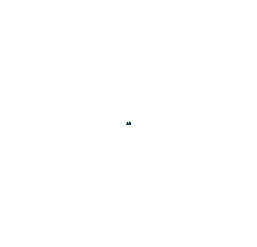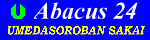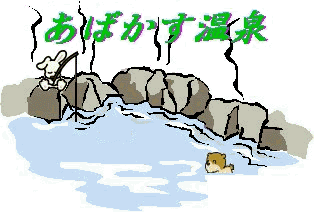丂丂丂杺曽恮Magic square

Magic square is a mathematical game which filling the square with the number from 1 in order to make the total amount of column,row,and the cross same.Use the same number of column and row. You can get the answer concening the odd number formation right here. You can find the way after trying some.Find out a rule of solving by yourself !!

[3乣23偺偆偪丄婏悢傪偊傜傫偱擖傟偰傒傛偆両]
Input an odd number from 3 to 23 !丂丂丂丂